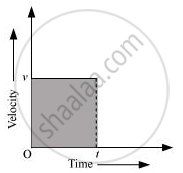# What is the quantity which is measured by the area occupied below the velocity-time graph? - Science

What is the quantity which is measured by the area occupied below the velocity-time graph?

#### Solution

DistanceThe graph shows the velocity-time graph of a uniformly moving body.

Let the velocity of the body at time (t) be v.

Area of the shaded region = length × breath

Where,

Length = t

Breath = v

Area = vt = velocity × time …(i)

We know,

"Velocity" = "Displacement"/"Time"

∴ Displacement = Velocity × Time…(ii)

From equations (i) and (ii),

Area = Displacement

Hence, the area occupied below the velocity-time graph measures the displacement covered by the body.

Concept: Graphical Representation of Linear Motion - Velocity - Time Graphs
Is there an error in this question or solution?

#### APPEARS IN

NCERT Class 9 Science
Chapter 8 Motion
Q 4 | Page 107